﻿ Fundamental properties of toroidal structures with current
 © Eugene A. Grigor'ev Fundamental properties of toroidal structures with current. The existence of an exterior magnetic field and structure of an interior magnetic field in toroidal structures with a poloidal current is shown.

In article "Maxwell's equations" was shown, that the closed electrical circuit, which use in the method of creation of a constant magnetic field by a separate element of a current, can be transformed to torus.
The numerical calculations of a magnetic field (MF) of toroidal structures with a poloidal current (Fig. 1) are made.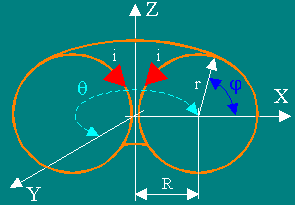Fig. 1

The arrows designated as i, shows vectors of current elements. The toruses with the relation R/r ≈ 1 and R/r ≈ 2 were considered. The outcomes of calculations are deduced as the graphs of the Cantor. The lines on the graphs display surfaces of a level of equal magnetic intensity.
Direction of a vector of a magnetic intensity - perpendicularly to a plane of the image, because the force lines of MF have only azimuthal (or tangential) component.

In the beginning was calculated a MF inside a torus.
Torus with the relation R/r ≈ 1(see. Fig. 1).Fig. 2

Torus with the relation R/r ≈ 2 (Fig. 3).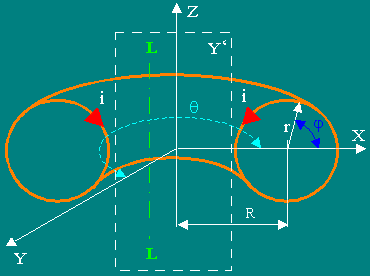Fig. 3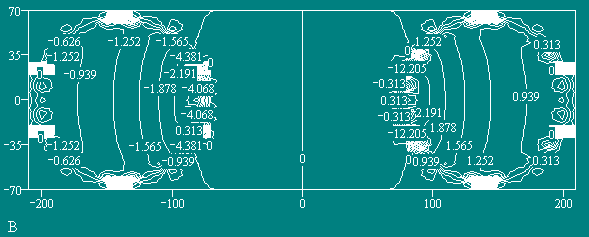Fig. 4

On the graphs (Fig. 2, the Fig. 4) is visible, that the structure of MF inside a torus does not correspond to structure of MF of an infinite direct conductor with a current, as was considered till now in the classical theory of an electromagnetism. This structure of MF corresponds to a field created by a separate part of a current located in centre of a torus on its principal axis and directional along this axis. The graph of it a MF is shown on a Fig. 5.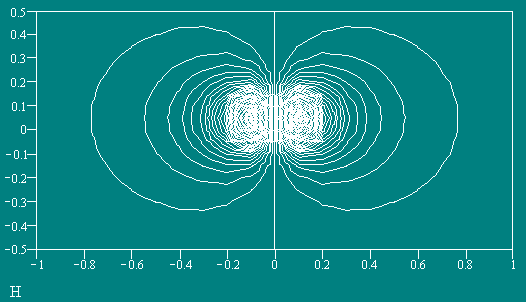Fig. 5

Then was calculated MF outside of a torus in a plane XZ (Y=0) in its part Y' ( Fig. 3).Fig. 6
Strength of MF in a plane Y ' (y=0) as the graph of the Cantor.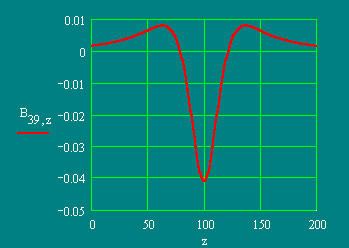Fig. 7
Strength of MF along direct L - L; [y = 0, x = const, B = f (z)].

On the graph (Fig. 6) it is visible, that a MF outside of a torus is real. The graph in a Fig. 7 determines a singularity of it MF - three maximums and two a zeros. From this fact the two possible variants of interaction of two toruses at their axial approach follows: 1) in the beginning there is their pushing away, and after overcoming a potential barrier - attraction; 2) in the beginning attraction, and then stabilization on some distance. In both cases the system passes to the condition with the minimal magnetic flow (with the minimal energy) and becomes steady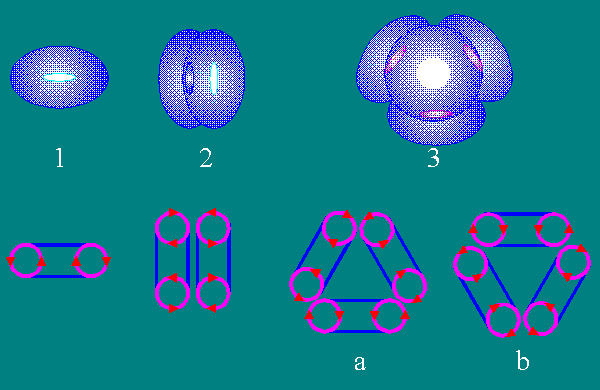Possible variants of interaction of toruses.
Quite probable mechanism of nucleogenesis from toroidal structures.

The calculation of MF, created by a system from two coaxial toruses (Fig. 8) show that it MF has a minimum on three coordinates at centre of a system (Fig. 9). It displays hopelessness of a plasma confinement in the closed traps with a toroidal configuration MF such as "Tokamak" and "Stellarator" by inside field of a torus - confinement probably only by outside field of a system of toruses of an arbitrary configuration.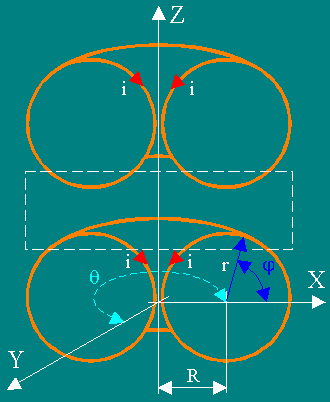Fig. 8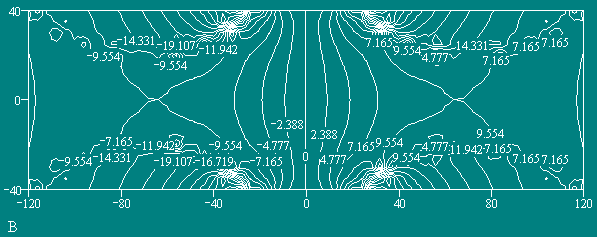Fig. 9

The previous calculations were made for continuous surfaces of current. Now we shall make calculation for a torus consisting of separate rectangular coils with a current (a segmented torus) (Fig. 10). It is done for considering of a possibility of reproduction MF of a continuous torus by a field of segmented (real) toruses.       The graphs - in relative units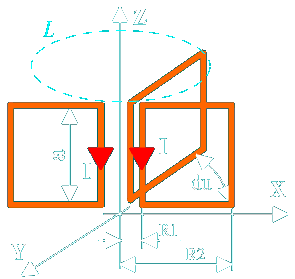Fig. 10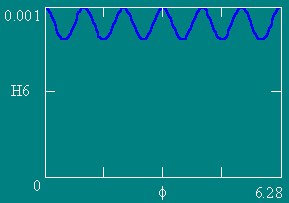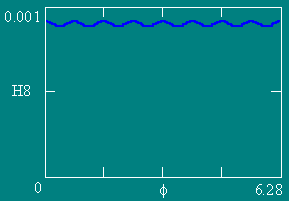The graphs of association of strength of MF (H) on a contour L (Z=0.1) from an angle f.
The number of coils NW is equal to 4, 6, 8 and 12 accordingly. Ampere-turns are constant.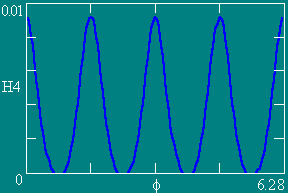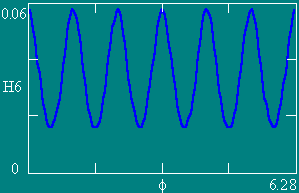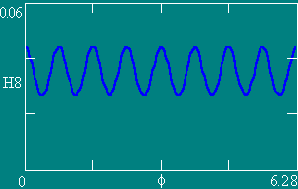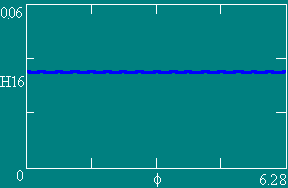The graphs of association of strength of MF (H) on a contour L (Z=0.05) from an angle f.
The number of coils NW is equal to 4, 6, 8 and 12 accordingly. Ampere-turns are constant.

On these two series of the graphs it is visible, that the force line of MF above the segmented torus is a rotationally symmetric circle with undulating axial component. In accordance with magnification of number of coils and distance from a torus a line more and more comes nearer to the shape of a force line of MF, created by continuous toroidal current surface - ideal circle.
The coil can be structural and consist of several conductors.

The graphs relating to a eight-coils segmented torus, are confirmed by experimental measuring!

These measuring have confirmed an existence of calculated exterior and interior structure of MF in toroidal formations with a poloidal current.
Like, similar to the external magnetic field is detected experimentally.
In experiment the arbitrary closed contour L which is not enveloping of currents but in which the circulation of a vector of a magnetic intensity is not equal to a zero is found.

The calculations for the toruses with other geometry are shown.